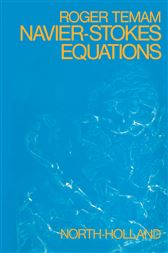Navier—Stokes Equations (2nd ed.)

Theory and Numerical AnalysisCA\$104.00
ISBNs
• 9780444853073
• 9781483256856

Navier-Stokes Equations: Theory and Numerical Analysis focuses on the processes, methodologies, principles, and approaches involved in Navier-Stokes equations, computational fluid dynamics (CFD), and mathematical analysis to which CFD is grounded.

The publication first takes a look at steady-state Stokes equations and steady-state Navier-Stokes equations. Topics include bifurcation theory and non-uniqueness results, discrete inequalities and compactness theorems, existence and uniqueness theorems, discretization of Stokes equations, existence and uniqueness for the Stokes equations, and function spaces. The text then examines the evolution of Navier-Stokes equations, including linear case, compactness theorems, alternate proof of existence by semi-discretization, and discretization of the Navier-Stokes equations. The book ponders on the approximation of the Navier-Stokes equations by the projection and compressibility methods; properties of the curl operator and application to the steady-state Navier-Stokes equations; and implementation of non-conforming linear finite elements.

The publication is a valuable reference for researchers interested in the theory and numerical analysis of Navier-Stokes equations.

• Elsevier Science; June 2016
• ISBN: 9781483256856
• Edition: 2